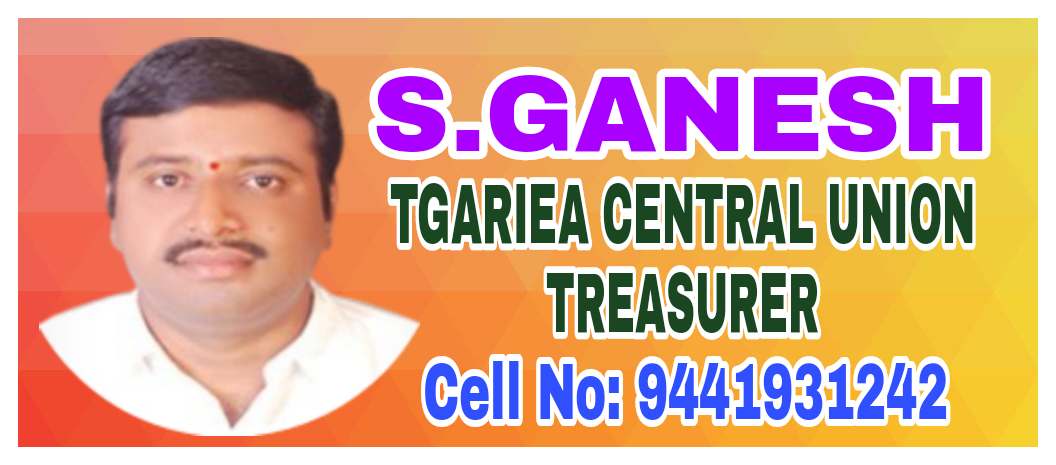# Probability Solution of TS & AP Board Class 9 Mathematics

###### Exercise 14.1

Question 1.

A die has six faces numbered from 1 to 6. It is rolled and number on the top face is noted. When this is treated as a random trial.

a) What are the possible outcomes?

b) Are they equally likely? Why?

c) Find the probability of a composite number turning up on the top face.

Here the sample space consist of outcomes = 1,2,3,4,5 and 6

Number of possible outcomes = 6

a) As sample space consist of all possible outcomes,

S (possible outcomes ) = 1,2,3,4,5 and 6

b) Yes, since every number (face) has equal possibility of coming up in a random trial they are equally likely. The possibility of each number is.

c) let E be the event of composite number turning up

the possible outcomes are 4 and 6

number of possible outcomes = 2

probability of a composite number turning up is =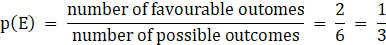Question 2.

A coin is tossed 100 times and the following outcomes are recorded

Tails:55 times from the experiment

a) Compute the probability of each outcomes.

b) Find the sum of probabilities of all outcomes.

Given that the coin is tossed 100 times, so sample space consist of 100 possible outcomes

Tails turns up 55 times

a) let E be the event of head turning up

Then P (E) =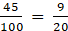Let F be event of tails turning up

P (F) =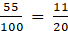b) sum of possibility of outcome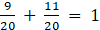Question 3.

A spinner has four colours as shown in the figure. When we spin it once, find

a) At which colour, is the pointer more likely to stop?

b) At which colour, is the pointer less likely to stop?

c) At which colours, is the pointer equally likely to stop?

d) What is the chance the pointer will stop on white?

e) Is there any colour at which the pointer certainly stops?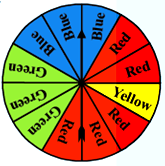a) The maximum number of partition on the spinner is of colors Red so the pointer is more likely to stop at color RED.

b) Color Yellow has only one partition so it is most likely to stop at it the least.

c) Since blue and green colors have equal partition on the spinner, it is more likely to stop on these two colors equally likely.

d) There is no white color partition on the wheel so there is no chance of spinner stopping on white color.

e) No, since it’s a random experiment all the colors have equal chance of being pointed by the spinner.

Question 4.

A bag contains five green marbles, three blue marbles, two red marbles, and two yellow marbles. One marble is drawn out randomly.

a) Are the four different colour outcomes equally likely? Explain.

b) Find the probability of drawing each colour marble i.e. , P(green), P(blue), P(red) and P(yellow)

c) Find the sum of their probabilities.

Here, sample space consists of 5 green marbles, three blue marbles, two red marbles and two yellow marbles. Total number of marbles are = 5 + 3 + 2 + 2 = 12

a) No, the four different color outcomes are not equally likely as green color marbles are maximum in number so the probability of drawing green color marble is most followed by blue color marbles.

b) Let G be the event of drawing green marble

Then P (G) =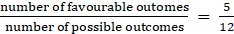Let B be the event of drawing blue marble

Then P (B) =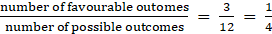Let R be the event of drawing a red marble

Then P (R) =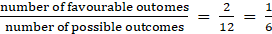Let Y be the event of drawing a yellow marble

Then P(Y) =(c) sum of all of the probabilities is =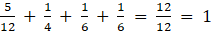Question 5.

A letter is chosen from English alphabet. Find the probability of the letters being

a) A vowel

b) a letter comes after P

c) A vowel or a consonant

d) Not a vowel

Here sample space consist of 26 alphabets. So total number of possible outcomes = 26

Out of these a, e, i , o and u are vowels , ∴ number of vowels are 5

And remaining 21 alphabets are consonants.

a) let E be the event of drawing a vowel

then, P (E) =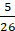b) There are q, r, s ,t , u, v, w , x ,y, z alphabets which comes after letter P.

The number of alphabets to come after p = 10

And let F be the event of drawing a letter which comes after P

P (F) =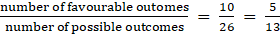(c) Since English alphabets consist of vowels or a consonant

The possibility of drawing a vowel or a consonant will be sum of probability of both the events happening equally likely

⇒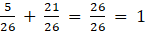Which is also the probability of a sure event.

(d) the consonant in English alphabets are b, c, d , f, g, h, , j , k ,l , m ,n, p, q , r ,s ,t ,v, w, x, y, z

The number of consonant are = 21

Let E be the event of drawing a consonant

Then P ( E) =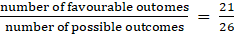Question 6.

Eleven bags of wheat flour, each marked 5 kg, actually contained the following weights of flour (in kg):

4.97, 5.05, 5.08, 5.03, 5.00, 5.06, 5.08, 4.98, 5.04, 5.07, 5.00

Find the probability that any of these bags chosen at random contains more than 5 kg of flour.

Here, the sample space is 11 as the total number of bags

being the same.

Let E be the event of choosing the bag with weight more than 5 kg

These bags have weight = 5.05, 5.08, 5.03, 5.06, 5.08, 5.04, 5.07

Hence Number of favorable outcomes = 7

∴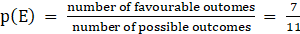Question 7.

An insurance company selected 2000 drivers at random (i.e., without any preference of one driver over another) in a particular city to find a relationship between age and accidents. The data obtained is given in the following table: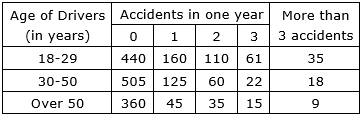Find the probabilities of the following events for a driver chosen at random from the city:

i. The driver being in the age group 18-29 years and having exactly 3 accidents in one year.

ii. The driver being in the age group of 30-50 years and having one or more accidents in a year.

iii. Having no accidents in the year.

here, sample space = 2000 being drivers selected at random

i) Given that the driver belongs to the age group of 18-29 and have exactly 3 accidents in one year

Let E be the event of selecting the driver from this age group with exactly having 3 accidents in a year

So the possible outcomes will consist 61

∴ P ( E) =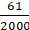ii.) Here the chances of choosing a driver from age group of

30 -50 and having 1 or more than 1 accident in a year will be total number of drivers meeting 1 or more than 1 accidents in a year

⇒ 125 + 60 + 22 + 18 = 225

Let E be the event of choosing the driver from this age group meeting more than 1 accident in a year

∴ P (E) =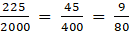iii.) let E be the event of choosing a driver who haven’t meet any accident in a year

The age group Is not mentioned so we will add all the age group drivers who haven’t meet any accident in the year

The number of drivers (favorable outcome)

= 440 + 505 + 360 = 1305

∴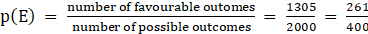Question 8.

What is the probability that a randomly thrown dart that hits the square board in shaded region. (side of square = 4cm)

(Take π = 22/7 and express in percentage)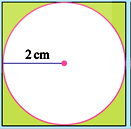Here, according to the given condition

Area of the square = side2 = 4 × 4 = 16 cm2

This is also the possible outcome

Area of the circle = π r2 =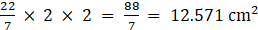Area of the shaded region = area of square – area of circle

= 16 – 12.57 = 3.428 cm2

which is also a favorable outcome

let E be the event of dart hitting the shaded region

∴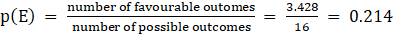= 21.4%

## MATHS VIDEOS